#### 期刊菜单

Application of TEM and Radioactivity Exploration in Deep Underground Water Resource Exploration
DOI: 10.12677/GST.2020.81005, PDF , HTML, XML, 下载: 593  浏览: 1,128

Abstract: The large loop source centered transient electromagnetic method is widely used in the exploration of deep groundwater resources. The calculation method of the late apparent resistivity adopted by TEM has obvious boundary effect. In order to suppress the boundary effect effectively, the method of calculating the all-time apparent resistivity is adopted in this paper. In order to solve the problem of serious shortage of water resources in a certain area of Shaanxi Province, TEM and radiometric methods were used to survey the groundwater resources in this area. The location of underground water bearing fracture can be roughly determined by the distribution of apparent resistivity, the range of fracture structure can be delineated by radioactive α-ray anomaly, and the apparent resistivity and radioactive α-ray anomaly measurement can reflect each other to improve the measurement accuracy. This work has reached the predetermined goal, and the newly determined four water wells meet the design requirements of single well water spray volume, which further verifies the reliability of the comprehensive application of TEM and radioactive exploration in the exploration of deep groundwater resources.

1. 引言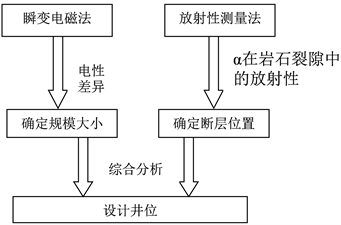Figure 1. Schematic diagram

2. 工作原理简介

2.1. TEM原理及全区视电阻率定义

$\rho \text{=}\frac{{\mu }_{0}}{4\text{π}t}{\left[2\text{π}{I}_{0}{a}^{2}{\mu }_{0}/\left(5t\frac{\epsilon \left(t\right)}{ns}\right)\right]}^{2/3}$

${H}_{Z}{\left(t\right)}_{回线}=\underset{i=1}{\overset{4}{\sum }}{\int }_{0}^{{L}_{i}}\frac{{I}_{y}}{4\text{π}{r}^{3}}\left[\left(1-\frac{3}{2{u}^{2}}\right)erf\left(u\right)+\frac{3}{u\sqrt{\text{π}}}\cdot {e}^{-{u}^{2}/2}\right]\text{d}L$

${H}_{Z}\left(t\right)=\frac{1}{sn{\mu }_{0}}\underset{0}{\overset{t}{\int }}{V}_{z}\left(t\right)\text{d}L$

${h}_{\tau }={\left(\frac{3Aq}{16\text{π}\left(v\left(t\right)/I\right){S}_{\tau }}\right)}^{1/4}-\frac{t}{{u}_{0}{S}_{\tau }}$

${S}_{\tau }=\frac{16{\text{π}}^{1/3}}{{\left(3Aq\right)}^{1/3}{u}_{0}^{4/3}}\frac{{\left(V\left(t\right)/I\right)}^{5/3}}{{\left(\text{d}\left(V\left(t\right)/I\right)/\text{d}t\right)}^{4/3}}$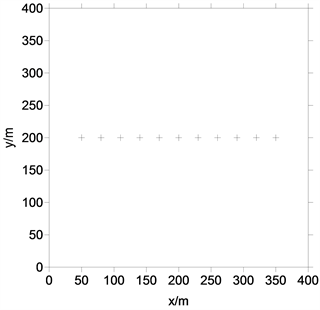Figure 2. Schematic of transmitter loop and measuring point position for forward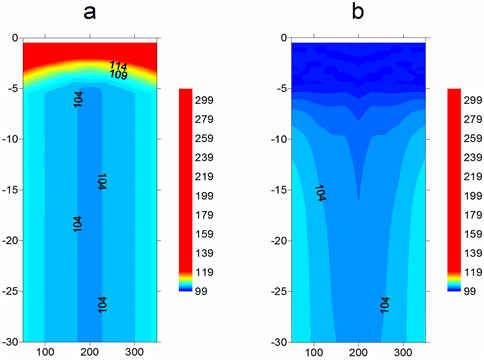Figure 3. Profile of apparent resistivity of model. (a) Later-time apparent resistivity; (b) All-time apparent resistivity

2.2. 应用放射性方法寻找地下水原理

1) 不同岩石拥有的放射性矿物的种类和含量都不相同；

2) 由于地质构造运动造成岩石挤压破碎变形，所以在构造及裂隙发育带中岩石的射气系数(即1克岩石在完全达到放射性平衡的时间内放出的氡量)具有更强的迁移能力，射气更易于到达地表，产生异常也更明显；

3) 地下水的地球化学作用导致土壤及岩石中的放射性元素发产生变化，出现放射性异常 。地下水分为孔隙裂隙水、土壤水以及不同层位间的层间水。孔隙裂隙水与成岩裂隙、风化裂隙以及构造裂隙等各种裂隙有紧密关系，其中构造裂隙带一般都是地下水储运都极为丰富的地带 。

3. 工区概况

3.1. 区内地质

3.2. 区内水文地质

3.2.1. 松散沉积层孔隙裂隙水

1) 下中更新统黄土状土潜水含水岩组

2) 下中更新统砂砾石状潜水含水岩组

3) 上新统承压水含水岩组

3.2.2. 基岩裂隙水

1) 三迭系下中统纸坊群裂隙水含水岩组

2) 二迭系上统石千峰组第三段裂隙水含水岩组

4. 数据采集及资料处理

4.1. 数据采集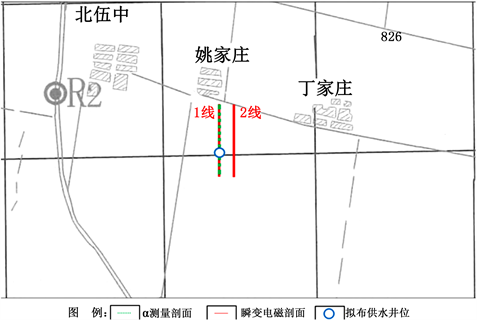Figure 4. Measuring point floorplan

4.2. 资料处理

5. 资料解释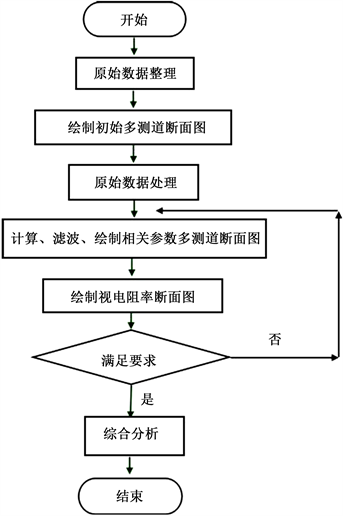Figure 5. Data processing flow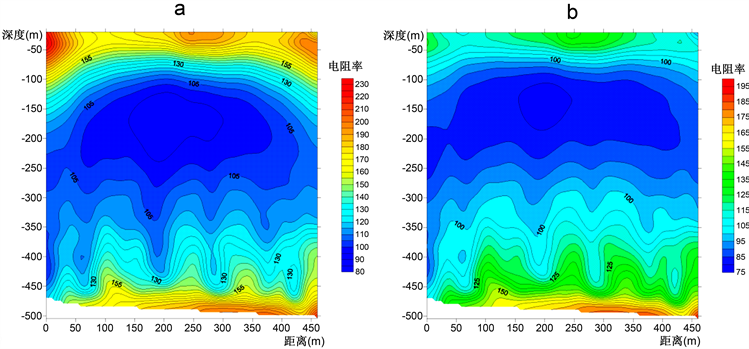Figure 6. Profile of apparent resistivity of measured data. (a) Later-time apparent resistivity; (b) All-time apparent resistivity

5.1. 资料解释Figure 7. The apparent resistivity section, radioactive α curve and well location design

5.2. 钻孔验证

6. 结论

1) TEM技术在寻找地下水资源时具有以下优势：① 勘察深度大；② 分辨率高；③ 效果显著等，能够有效提高找水的工作效率。

2) 全区视电阻率计算的方法能够有效规避晚期视电阻率计算方法所存在的“边界效应”，避免了早期道的高值假异常，能够更加真实的反映出模型的地电情况，更利于大回线源瞬变电磁法所采集数据的处理和后期解译，对于进一步提高工程物探的精准度，有着积极意义。

3) 工程物探中一般依据电阻率越低富水可能性越高的原则，但多种因素都可能对电阻率产生影响，且物探本身具有多解性，复杂的地质条件也可能对测量数据产生影响，可能导致最终的推测结果与实际情况有所偏差。结合放射性测量，可以有效避免上述情况，两种方法结合，避免一种方法的缺陷，发挥各自的优点，以相互验证、补充，有效的避免的单一方法的多解性，进一步降低工程物探的风险，工作效率有了显著提升。

  李茂榕, 王平. 西部地下水资源勘查的综合地球物理问题——以鄂尔多斯盆地白垩系地下水勘查为例[J]. 地球物理学进展, 2001, 16(3): 119-126.  潘玉玲, 张昌达. 地面核磁共振找水理论和方法[M]. 武汉: 中国地质大学出版社, 2000: 98-107.  梁建刚, 刘黎东, 高学生, 等. 电阻率法和激发极化法在地下水勘察中的应用[J]. 物探与化探计算技术, 2014, 36(4): 415-417.  吴小洁, 张前, 陈长亮, 等. 综合电法勘探在五指山地区找热矿水中的应用[J]. 工程地球物理学报, 2015, 12(3): 348-350.  张彪, 刘良志, 倪进鑫, 等. 综合物探方法在花岗岩严重缺水地区找水勘察中的应用[J]. 工程地球物理学报, 2015, 12(4): 501-505.  李金铭, 程学栋, 等. 激电找水应用基础研究[J]. 物探与化探, 1990, 14(4): 273-274.  冯兵, 孟小红, 张斌. TEM框内回线装置发射框边界影响及消除方法[J]. 煤田地质与勘探, 2010, 38(5): 61-66.  邹力, 葛君伟, 方方, 唐红, 贾文懿. 静电卡法在贵州普定找寻水库漏水处及地下暗河的实验[J]. 成都地质学院学报, 1985, 8(3): 93-94.  程业勋. 核辐射场与放射性勘查[M]. 第1版. 北京: 地质出版社, 2005.